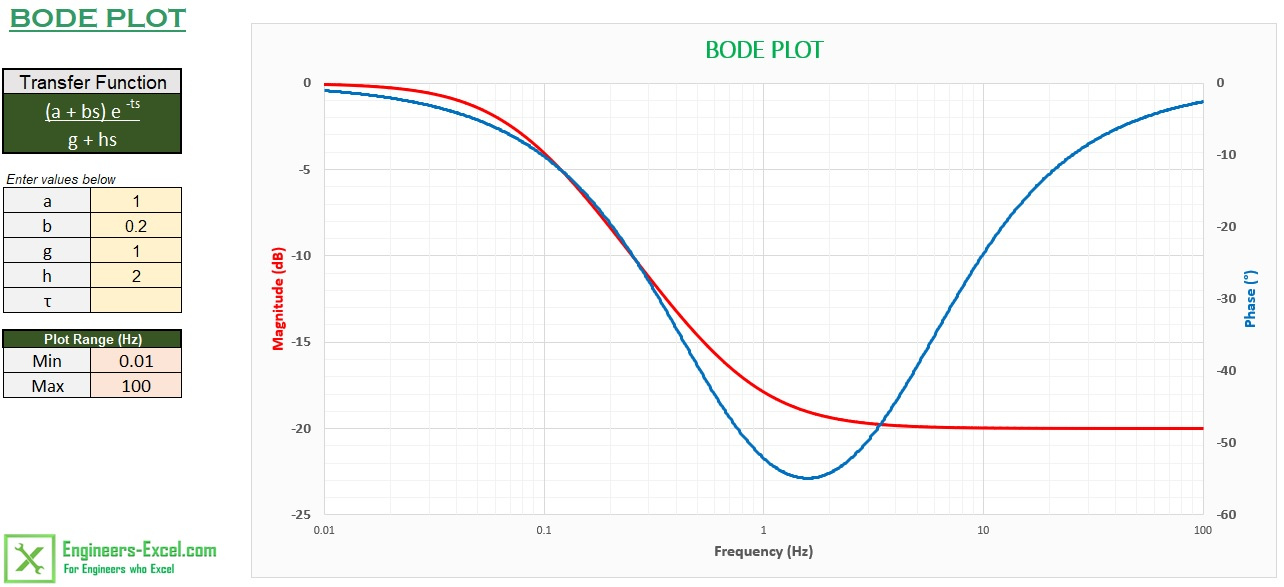# Bode Plot Generator

This app will generate the Bode Plot given a transfer function of the form (a + bs) e-ts / (g + hs).For generating the Bode plot for a system of a higher order, see Bode Plot using Complex Functions.

.xlsx file

## How to use

The use of this app is quite straightforward, simply key in the values of a, b, g, h and t into the sheet, along with the frequency range for the plot. Note that the units of frequency is rad/time.

Lets say we wish to obtain the Bode plot of (1 + 0.2s)/(1 + 2s), from 0.01 rad/s to 100 rad/s. We have
a = 1,
b = 0.2,
g =1,
h = 2 and
t = 0.

Key in these values and the range. If done correctly, you should see the screen below.## Analysis of signal filters using Bode Plots

Bode Plots are used to study the frequency response of signal filters. Lets use the Bode Plot tool to study the frequency response of a low pass filter. A low pass filter allows low frequencies to pass through but attenuates higher frequencies.

Consider a low pass filter made from a resistor and capacitor:The transfter function for this filter is given by

TF = 1 / (1 + RCs)

Lets look at the frequency response when R = 10 kohms and C = 2 microfarad. Here, we have a = 1, b = 0, g = 1, h = 0.02 and t = 0. From the Bode plot, we see that this filter has a cut off frequency of 50 rad/s or 7.96 Hz.## How it works

The amplitude and phase are calculated at 1000 points over the frequency range using defined names and formulae. All formulae are evaluated in the memory, there are no calculations done directly on the spreadsheet.

Note that the division of the frequency is done on a logarithmic scale. The formula for the frequency is:

w=10^(LOG(Sheet1!\$B\$13)+(ROW(OFFSET(Sheet1!

\$A\$1,0,0,1001,1))-1)*(LOG(Sheet1!\$B\$14)-LOG(Sheet1!\$B\$13))/1000)

The ROW and OFFSET formulae are used to generate the array of numbers 1 to 1000, which is then used to get 1000 points between the specified range. See page 531 of the sample chapter 15 from Stephen Bullen's book Professional Excel Development for a detailed description of this technique.

The amplitude calculation is quite straightforward, the formula is

Amplitude = SQRT((a^2+(b*w)^2)/(g^2+(h*w)^2)),

which is the ratio of the magnitude of the numerator divided by the magnitude of the denominator.

The calculation for the phase angle is not so straightforward:

Phase = IF(ATAN2(a,b*w)<0,360+DEGREES(ATAN2(a,b*w)),DEGREES(ATAN2(a,b*w)))-IF(ATAN2(g,h*w)<0, 360+DEGREES(ATAN2(g,h*w)),DEGREES(ATAN2(g,h*w)))+DEGREES(-t*w)

In simple words, the formula is Phase = (Phase of Numerator) - (Phase of denominator) + (Phase from the exp term).

The calculation uses the ATAN2 formula, which gives the inverse tangent of the specified x- and y-coordinates between -PI and PI. Since this range is not applicable for the Bode plot, it is modified using the IF statement. The angles are converted to degrees using the DEGREES formula.

## Also See:

Nyquist Plot GeneratorZiegler Nichols Tuning Calculator